Aniruddha Bhandari — Published On June 16, 2020 and Last Modified On February 8th, 2023

## Introduction

You’ve built your machine learning model – so what’s next? You need to evaluate and validate how good (or bad) it is, so you can decide whether to implement it. That’s where the AUC-ROC curve comes in.

The name might be a mouthful, but it is just saying that we are calculating the “Area Under the Curve” (AUC) of the “Receiver Operating Characteristic” (ROC). Confused? I feel you! I have been in your shoes. But don’t worry, we will see what these terms mean in detail, and everything will be a piece of cake!For now, just know that the AUC-ROC curve helps us visualize how well our machine learning classifier performs. Although it works only for binary classification problems, we will see how we can extend it to evaluate multi-class classification problems.

We’ll also cover topics like sensitivity and specificity since these are key topics behind the AUC-ROC curve (or ROC AUC curve in machine learning).

I suggest going through the article on Confusion Matrix as it will introduce some important terms we will use in this article.

## What are Sensitivity and Specificity?

This is what a confusion matrix looks like: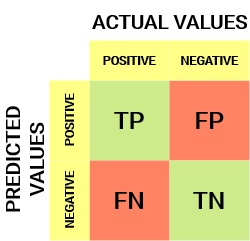From the confusion matrix, we can derive some important metrics that were not discussed in the previous article. Let’s talk about them here.

#### Sensitivity / True Positive Rate / Recall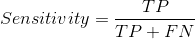Sensitivity tells us what proportion of the positive class got correctly classified.

A simple example would be determining what proportion of the actual sick people were correctly detected by the model.

#### False Negative Rate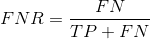False Negative Rate (FNR) tells us what proportion of the positive class got incorrectly classified by the classifier.

A higher TPR and a lower FNR are desirable since we want to classify the positive class correctly.

#### Specificity / True Negative Rate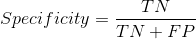Specificity tells us what proportion of the negative class got correctly classified.

Taking the same example as in Sensitivity, Specificity would mean determining the proportion of healthy people who were correctly identified by the model.

#### False Positive Rate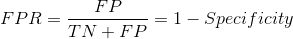FPR tells us what proportion of the negative class got incorrectly classified by the classifier.

A higher TNR and a lower FPR are desirable since we want to classify the negative class correctly.

Out of these metrics, Sensitivity and Specificity are perhaps the most important, and we will see later on how these are used to build an evaluation metric. But before that, let’s understand why the probability of prediction is better than predicting the target class directly.

## Probability of Predictions

A machine learning classification model can be used to directly predict the data point’s actual class or predict its probability of belonging to different classes. The latter gives us more control over the result. We can determine our own threshold to interpret the result of the classifier. This is sometimes more prudent than just building a completely new model!

Setting different thresholds for classifying positive classes for data points will inadvertently change the Sensitivity and Specificity of the model. And one of these thresholds will probably give a better result than the others, depending on whether we are aiming to lower the number of False Negatives or False Positives.

Have a look at the table below: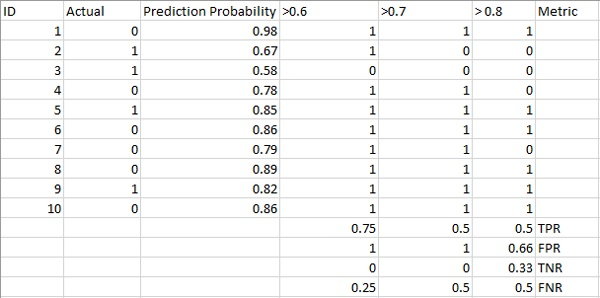The metrics change with the changing threshold values. We can generate different confusion matrices and compare the various metrics that we discussed in the previous section. But that would not be a prudent thing to do. Instead, we can plot roc curves between some of these metrics to quickly visualize which threshold is giving us a better result.

The AUC-ROC curve solves just that problem!

## What is the AUC-ROC Curve?

You might have come across the terms ROC and AUC associated with artificial intelligence, deep learning, and convolutional neural networks (CNNs). So what exactly are they?

The Receiver Operator Characteristic (ROC) curve is an evaluation metric for binary classification problems. It is a probability curve that plots the TPR against FPR at various threshold values and essentially separates the ‘signal’ from the ‘noise.’ In other words, it shows the performance of a classification model at all classification thresholds. The Area Under the Curve (AUC) is the measure of the ability of a binary classifier to distinguish between classes and is used as a summary of the ROC curve.

The higher the AUC, the better the model’s performance at distinguishing between the positive and negative classes.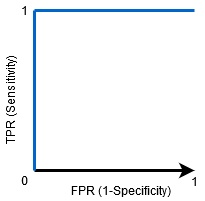When AUC = 1, the classifier can correctly distinguish between all the Positive and the Negative class points. If, however, the AUC had been 0, then the classifier would predict all Negatives as Positives and all Positives as Negatives.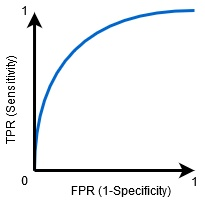When 0.5<AUC<1, there is a high chance that the classifier will be able to distinguish the positive class values from the negative ones. This is so because the classifier is able to detect more numbers of True positives and True negatives than False negatives and False positives.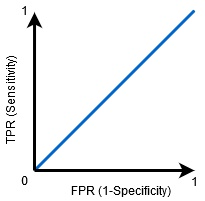When AUC=0.5, then the classifier is not able to distinguish between Positive and Negative class points. Meaning that the classifier either predicts a random class or a constant class for all the data points.

So, the higher the AUC value for a classifier, the better its ability to distinguish between positive and negative classes.

## How Does the AUC-ROC Curve Work?

In a ROC curve, a higher X-axis value indicates a higher number of False positives than True negatives. While a higher Y-axis value indicates a higher number of True positives than False negatives. So, the choice of the threshold depends on the ability to balance False positives and False negatives.

Let’s dig a bit deeper and understand what our ROC curve would look like for different threshold values and how the specificity and sensitivity would vary.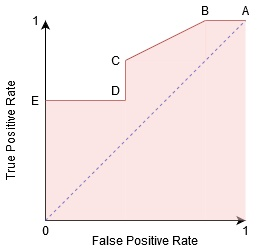We can try and understand this graph by generating a confusion matrix for each point corresponding to a threshold and talk about the performance of our classifier: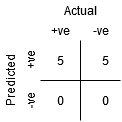Point A is where the Sensitivity is the highest and Specificity the lowest. This means all the Positive class points are classified correctly, and all the Negative class points are classified incorrectly.

In fact, any point on the blue line corresponds to a situation where the True Positive Rate is equal to False Positive Rate.

All points above this line correspond to the situation where the proportion of correctly classified points belonging to the Positive class is greater than the proportion of incorrectly classified points belonging to the Negative class.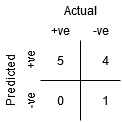Although Point B has the same Sensitivity as Point A, it has a higher Specificity. Meaning the number of incorrectly Negative class points is lower than the previous threshold. This indicates that this threshold is better than the previous one.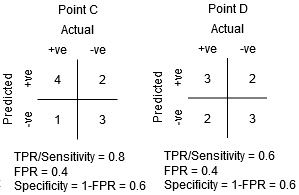Between points C and D, the Sensitivity at point C is higher than point D for the same Specificity. This means, for the same number of incorrectly classified Negative class points, the classifier predicted a higher number of Positive class points. Therefore, the threshold at point C is better than point D.

Now, depending on how many incorrectly classified points we want to tolerate for our classifier, we would choose between point B or C to predict whether you can defeat me in PUBG or not.

“False hopes are more dangerous than fears.”–J.R.R. Tolkein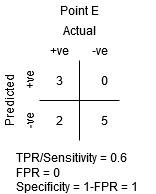Point E is where the Specificity becomes highest. Meaning the model classifies no False Positives. The model can correctly classify all the Negative class points! We would choose this point if our problem was to give perfect song recommendations to our users.

Going by this logic, can you guess where the point corresponding to a perfect classifier would lie on the graph?

Yes! It would be on the top-left corner of the ROC graph corresponding to the coordinate (0, 1) in the cartesian plane. Here, both the Sensitivity and Specificity would be the highest, and the classifier would correctly classify all the Positive and Negative class points.

## Understanding the AUC-ROC Curve in Python

Now, either we can manually test the Sensitivity and Specificity for every threshold or let sklearn do the job for us. We’re definitely going with the latter!

Let’s create our arbitrary data using the sklearn make_classification method:

Python Code:

I will test the performance of two classifiers on this dataset:

Sklearn has a very potent method, roc_curve(), which computes the ROC for your classifier in a matter of seconds! It returns the FPR, TPR, and threshold values:

The AUC score can be computed using the roc_auc_score() method of sklearn:

`0.9761029411764707 0.9233769727403157`

Try this code out in the live coding window below:

We can also plot the receiver operating characteristic curves for the two algorithms using matplotlib: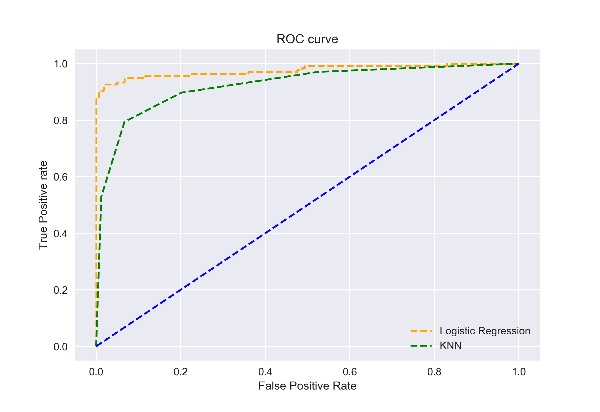It is evident from the plot that the AUC for the Logistic Regression ROC curve is higher than that for the KNN ROC curve. Therefore, we can say that logistic regression did a better job of classifying the positive class in the dataset.

## AUC-ROC Curve for Multi-Class Classification

As I said before, the AUC-ROC curve is only for binary classification problems. But we can extend it to multiclass classification problems using the One vs. All technique.

So, if we have three classes, 0, 1, and 2, the ROC for class 0 will be generated as classifying 0 against not 0, i.e., 1 and 2. The ROC for class 1 will be generated as classifying 1 against not 1, and so on.

The ROC curve for multi-class classification models can be determined as below: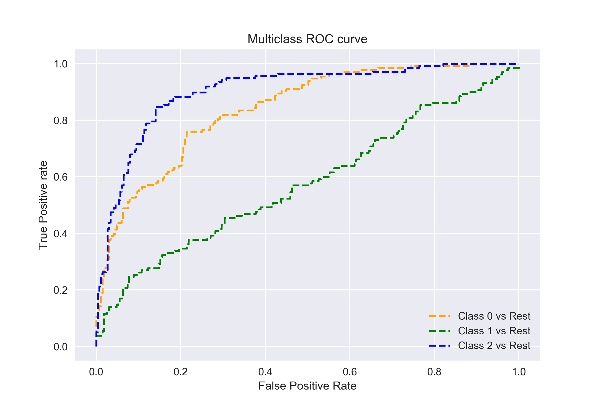## Conclusion

I hope you found this article helpful in understanding how powerful the AUC-ROC curve metric is in measuring the performance of a classifier. You’ll use this often in the industry and in data science or machine learning hackathons. Better get familiar with ROC AUC score, AUC formula machine learning, and ROC formula machine learning.

Going further, I would recommend you the following courses that will be useful in building your data science acumen:

#### Q1. What is the AUC ROC curve?

A. AUC ROC stands for “Area Under the Curve” of the “Receiver Operating Characteristic” curve. The AUC ROC curve is basically a way of measuring the performance of an ML model. AUC measures the ability of a binary classifier to distinguish between classes and is used as a summary of the ROC curve.

#### Q2. What does the ROC AUC score tell you?

A. The ROC AUC score tells us how efficient the model is. The higher the AUC, the better the model’s performance at distinguishing between the positive and negative classes. An AUC score of 1 means the classifier can perfectly distinguish between all the Positive and the Negative class points. An AUC value of 0 shows that the classifier predicts all Negatives as Positives and vice versa.

#### Q3. What does ROC AUC 0.5 mean?

A. ROC AUC 0.5 means that the classifier is not working. An AUC value above 0.5 means the classifier can detect more numbers of True positives and True negatives than False negatives and False positives.## 10 thoughts on "Guide to AUC ROC Curve in Machine Learning : What Is Specificity?"###### Ogah says:June 16, 2020 at 3:10 pm
Thanks. It a good write up Reply###### Jon T says:June 16, 2020 at 4:01 pm
Hi Aniruddha, Thank you for writing such a comprehensive overview of AUC-ROC metrics. I just have a query regarding your example table in the Probability of Predictions section: at the bottom of the table, why is FPR=0, TNR=1 rather than the other way around? Thanks Jon Reply###### Mahtab says:June 16, 2020 at 7:10 pm
Very grateful post for me, I want to say you tha k you so much Reply###### Yami says:June 16, 2020 at 10:27 pm
p_fpr, p_tpr, _ = roc_curve(y_test, random_probs, pos_label=1) What to use inside random_probs, as it is throwing error Reply###### Aniruddha Bhandari says:June 16, 2020 at 11:30 pm
Thanks, Jon for pointing it out. Reply###### Aniruddha Bhandari says:June 16, 2020 at 11:30 pm